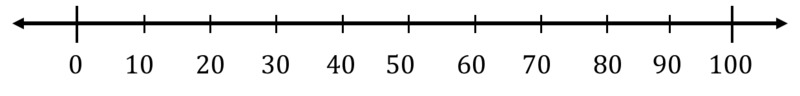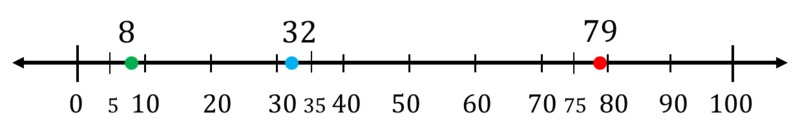# Rounding to the Nearest Ten and Hundred

Alignments to Content Standards: 3.NBT.A.1

Plot 8, 32, and 79 on the number line.1. Round each number to the nearest 10. How can you see this on the number line?

2. Round each number to the nearest 100. How can you see this on the number line?

## IM Commentary

In third grade, students "use place value understanding to round whole numbers to the nearest 10 or 100" (3.NBT.A.1). The purpose of this task is for students to practice rounding numbers to the nearest ten, connect the rules for rounding with location on the number line, and to introduce the idea of rounding to the nearest 100. This task naturally builds towards 3.NBT, 4.NBT Rounding to the Nearest 100 and 1000 which provides a transition between third and fourth grade rounding expectations since fourth graders are expected to "use place value understanding to round multi-digit whole numbers to any place" (4.NBT.A.3). That task, in turn, builds towards 4.NBT Rounding to the Nearest 1000.

## Solution

The three numbers are plotted on the number line below:For rounding to the nearest 10 we find which two tens the number lies between and round to the nearer of the two. Similarly, for rounding to the nearest 100, we find the two hundreds the number lies between (0 and 100 for all of these numbers) and round to the nearer of the two.

1. 8 lies between 0 and 10. 5 is exactly half-way between 0 and 10, and 8 is between 5 and 10. So 8 is closer to 10 than to 0 on the number line and so it rounds up to 10.

32 lies between 30 and 40. 35 is exactly half-way between 30 and 40, and 32 is between 30 and 35. So 32 is closer to 30 than 40 on the number line and so it rounds down to 30.

79 lies between 70 and 80. 75 is exactly half-way between 70 and 80, and 79 is between 75 and 80. So 79 is closer to 80 than 70 on the number line and it rounds up to 80.

2. 50 is exactly half-way between 0 and 100. 8 rounds down to 0 because it is closer to 0 than to 100 on the number line.

50 is exactly half-way between 0 and 100. 32 rounds down to 0 because it is closer to 0 than 100 on the number line.

50 is exactly half-way between 0 and 100. 79 rounds up to 100 because it is closer to 100 than 0 on the number line.